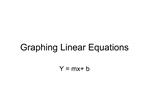DownloadDownload PresentationGraphing Linear Equations

# Graphing Linear Equations

Télécharger la présentation## Graphing Linear Equations

- - - - - - - - - - - - - - - - - - - - - - - - - - - E N D - - - - - - - - - - - - - - - - - - - - - - - - - - -
##### Presentation Transcript

1. Graphing Linear Equations Y = mx+ b

2. Calculator (set-up) Write equation in the form y = mx + b Press the Y= and type equation into Y1 Press ZOOM . Then go to (#6ZStandard) and press ENTER Note: if the graph does not cross the y axis then: Press TRACE and look at bottom values of X and Y. If the y value is greater than 10 or less than -10, change the WINDOW Press WINDOW. If the trace value of y is greater than 10 then increase the ymax so it is larger than the trace value. If the trace value of y is less than -10, then decrease the ymin so it is less than the trace value. Press GRAPH DONE